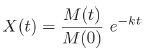Ecological Archives E093-014-A2

Etienne Laliberté and Jason M. Tylianakis. 2012. Cascading effects of long-term land-use changes on plant traits and ecosystem functioning. Ecology 93:145–155.

Appendix B.Details on the estimation of litter decomposition rates.

We used the negative exponential single-pool decomposition model (Olson 1963) to estimate the litter decomposition rates k:where X(t) is the proportion of litter mass remaining at time t (in days), M(t) is the litter mass remaining at time t, and M(0) is the initial litter mass. By definition, X(t) is in theory bounded between 0 and 1, although in practice values > 1 sometimes occur. The litter decomposition rates k were estimated via maximum likelihood (N = 20 for each of the 60 plot/incubation type combinations) on untransformed data, using the 'bbmle' package (Bolker 2010) in the R environment (R Development Core Team 2010).

Nonlinear regression on untransformed data with normal errors has recently been recommended for estimating decomposition rates in single-pool models (Adair et al. 2010). However, the beta distribution can provide a better alternative for modeling proportional bounded data, which often show reduced variance near the bounds (Smithson and Verkuilen 2006). Thus, we fitted models with either normal or beta errors and selected the best one based on ΔAICc (the change in residual deviance, measured using the Akaike Information Criterion for small sample sizes). Because the beta distribution only allows values from the open unit interval 0 < y < 1, whenever a value > 1 was found we first compressed those data to the closed unit interval [0, 1] by taking y' = y / a, where a is the maximum y value, and then compressing the range to the open unit interval ]0, 1[ by taking y'' = [y'(N - 1) + 0.5] / N, where N is the number of data points (Smithson and Verkuilen 2006). This transformation had very little effect on the data (results not shown).

Literature Cited

Adair, E. C., S. E. Hobbie, and R. K. Hobbie. 2010. Single-pool exponential decomposition models: potential pitfalls in their use in ecological studies. Ecology 91:1225–1236.

Bolker, B. M. 2010. bbmle: Tools for general maximum likelihood estimation. The Comprehensive R Archive Network (CRAN), Vienna, Austria.

Olson, J. S. 1963. Energy storage and the balance of producers and decomposers in ecological systems. Ecology 44:322–331.

R Development Core Team. 2010. R: A language and environment for statistical computing. R Foundation for Statistical Computing, Vienna, Austria.

Smithson, M., and J. Verkuilen. 2006. A better lemon squeezer? Maximum-likelihood regression with beta-distributed dependent variables. Psychological Methods 11:54–71.